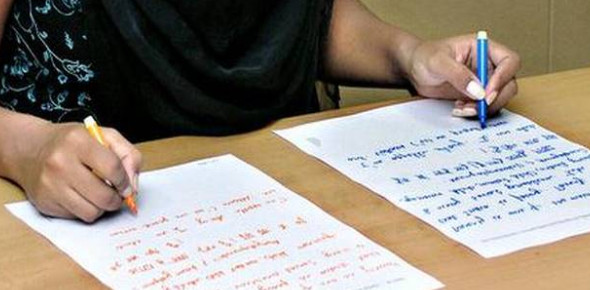# Are You A Lefty Or A Righty? Maybe Ambidextrous? Take This 60 Second Quiz To Find Out.

18 Questions | Total Attempts: 18000Determine how much of a lefty you are. Take this 60 second quiz to find out.

• 1.
Answer "left" or "right" for each activity in each question depending on which hand/side you use more often in that activity. Writing
• A.

Left

• B.

Right

• 2.
Drawing
• A.

Left

• B.

Right

• 3.
Throwing
• A.

Left

• B.

Right

• 4.
Scissors
• A.

Left

• B.

Right

• 5.
Combing
• A.

Left

• B.

Right

• 6.
• A.

Left

• B.

Right

• 7.
Knife without use of a fork
• A.

Left

• B.

Right

• 8.
Spoon
• A.

Left

• B.

Right

• 9.
Hammer
• A.

Left

• B.

Right

• 10.
Screwdriver
• A.

Left

• B.

Right

• 11.
Tennis
• A.

Left

• B.

Right

• 12.
Golf club
• A.

Left

• B.

Right

• 13.
Broom
• A.

Left

• B.

Right

• 14.
Rake
• A.

Left

• B.

Right

• 15.
Match - lighter
• A.

Left

• B.

Right

• 16.
Opening a jar lid
• A.

Left

• B.

Right

• 17.
Dealing Cards
• A.

Left

• B.

Right

• 18.
Foot ( which foot would you step forward with first when starting to walk?)
• A.

Left

• B.

Right

Related TopicsBack to top